# 1.     Background

The purpose of this article is to provide a way of showing different color in alternate rows in the report. It will make report more interactive and simplified the report for the business users. Here we are going to use SSRS function – RowNumber and Modulo function for alternate row coloring.

# 2.     What is RowNumber and Modulo function in SSRS?

• RowNumber Function:  returns a running value of the count of rows within the specified scope. Each row is having a count under a specified scope. Row/Column Groups are good example of scope. In the below example, we are using RowNumber (Nothing) as expression for a text box (row).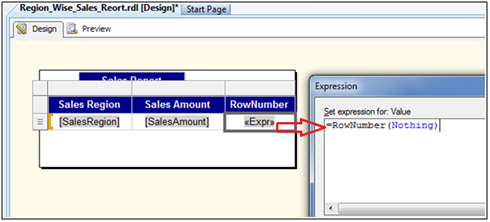It will return the below values for the rows: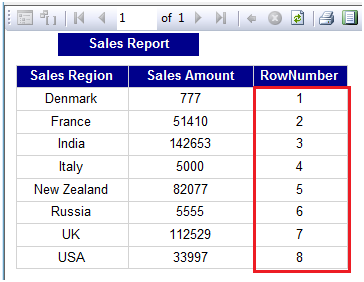• Modulo Function: Returns the remainder of one number divided by another.
Syntax: dividend % divisor

Below is the example showing dividend is divide by divisor and getting reminder as result:
SELECT
'1%2' AS [dividend % divisor] ,1%2 AS [Result]
SELECT '2%2' AS [dividend % divisor] ,2%2 AS [Result]
SELECT '3%2' AS [dividend % divisor], 3%2 AS [Result]
SELECT '4%2' AS [dividend % divisor],4%2 AS [Result]
SELECT '5%2' AS [dividend % divisor],5%2 AS [Result]
SELECT '6%2' AS [dividend % divisor],6%2 AS [Result]
SELECT '7%2' AS [dividend % divisor],7%2 AS [Result]
SELECT '8%2' AS [dividend % divisor],8%2 AS [Result]
SELECT '9%2' AS [dividend % divisor],9%2 AS [Result]
SELECT '10%2' AS [dividend % divisor],10%2 AS [Result]

It will return reminder as 1 or 0.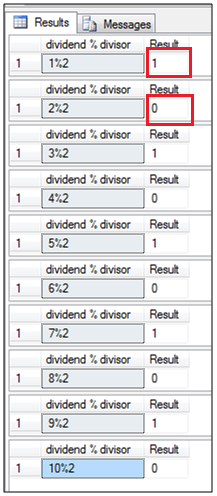• Final Expression : =IIF(RowNumber(Nothing) Mod 2 = 0,"Magenta","Silver")
It means every row count will be divided by 2 and reminder will be calculated as 1 or 0. If reminder is 0 then row should be displayed in “Magenta” color and if reminder is 1 then row should be displayed in “Silver” color.

# 3.     Steps to add alternating row color in the report:

Below is the simple Sales report in which we will implement alternate row coloring: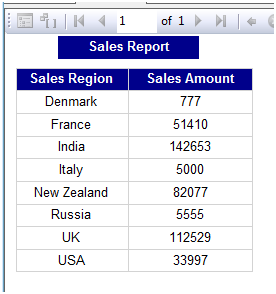I.            Select the rows in which alternate row color need to be implement: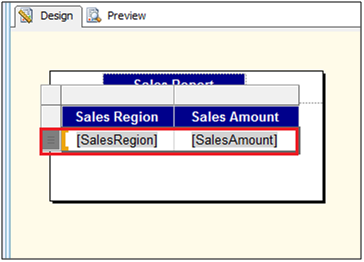II.            Go to the background property and select expression: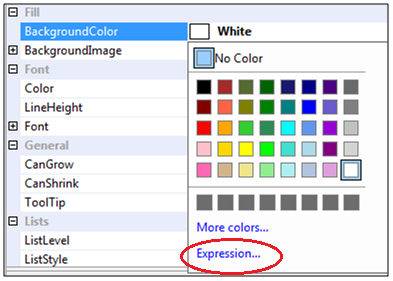III.            Write the below expression box and click ok.
=IIF (RowNumber (Nothing) Mod 2 = 0,"Magenta","Silver")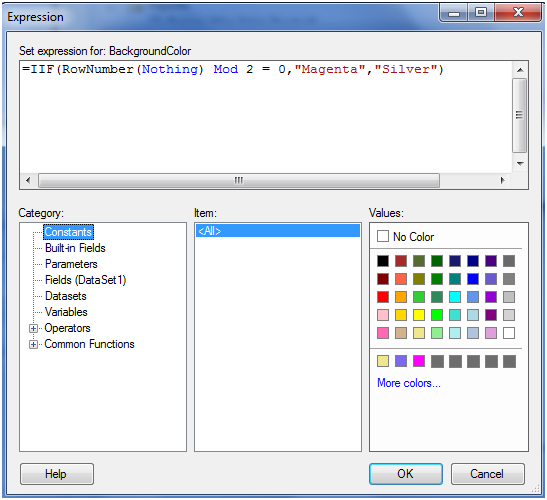IV.            Preview the report. We can see the alternate color rows.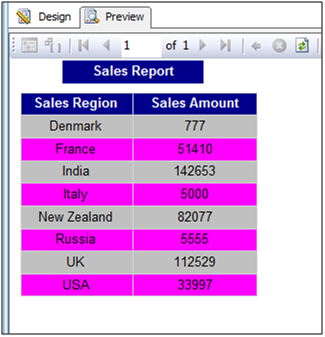# 4.     Conclusion

By using SSRS RowNumber and Modulo (MOD) function, we can implement coloring of alternate rows.
----------------------------------End of Document----------------------------------